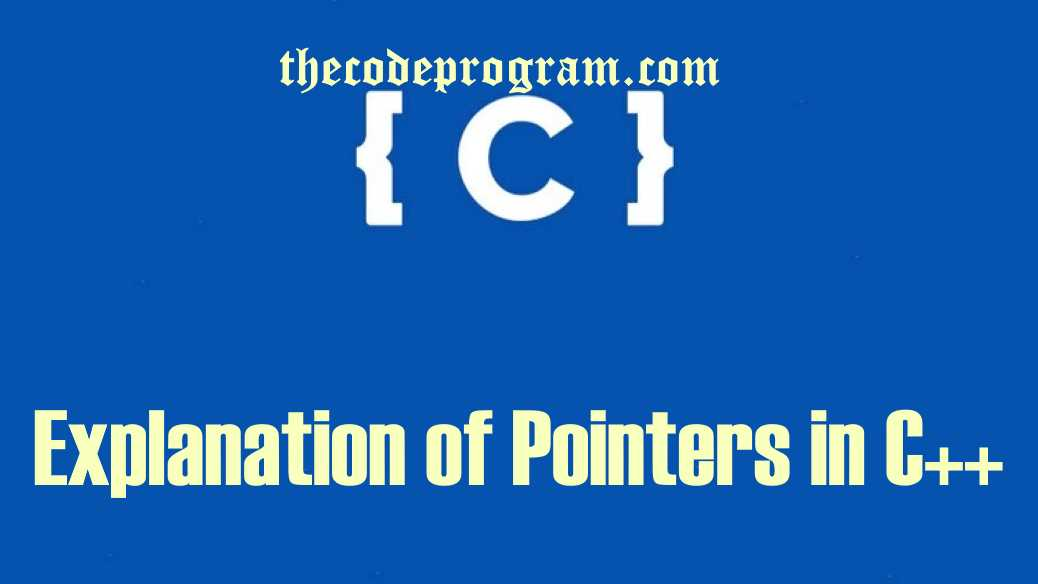## Explanation of Pointers in C++

Hello everyone, in this article we are going to see the pointers in C++. We will see the purpose of the pointers and what can we do with pointers with example in QT Console Application.

Let's Begin.

Declared every variable has an address value on the memory. Normally the developer do not do something with these aderesses. When the program started the variables being located at the memory automatically and CPU reach them via these addresses. Sometimes we developers may be in need of accessing directly these addresses of the variables. In these cases the pointers comes for our help.

We use the (&) operator to get the variable address.

``````
#include <QCoreApplication>
#include <iostream>
``````
``````
using namespace std;

int main(int argc, char *argv[])
{
QCoreApplication a(argc, argv);

int  var_1;
bool var_2;

cout << "Address of var_1 is " << &var_1 << endl ;
cout << "Address of var_2 is " << &var_2 << endl;

return a.exec();
}
``````

As you can see below progam output we have the adresses of the varables.

``````
``````

These values will be chenged at every program restarted. Because the program always be writed differant adresses on the memory.

#### So What are pointers?

Pointers are a variable types to keep the addresses of the variables. We declare the variables. We use the (*) operator to declare the pointers. Below you will how to declare a pointer:

``````
int  *p_int_var;   //an integer pointer
bool *p_bool_var;  //a bool pointer
``````

You can not assign a variable directly to these pointers even if same variable types. You can only assign the address values. When you try to get value of the pointer you will have the address of the pointer. If you want to get the variable value which assigned to the pointer by address you must use the unary operator(*).

You can see the example code below:

``````
cout << "Define and use the pointers : " << endl;

int  *p_int_var;   //an integer pointer
bool *p_bool_var;  //a bool pointer

var_1 = 33;
var_2 = true;

p_int_var = &var_1;
p_bool_var = &var_2;

cout << "Value of var_1: " << var_1 << ", Adress is : " << p_int_var << endl;
cout << "Value of var_2: " << var_2 << ", Adress is : " << p_bool_var << endl;

cout << "Address of p_int_var : " << p_int_var << " , value is :  " <<*p_int_var << endl;
cout << "Address of p_bool_var : " << p_bool_var << " , value is :  " <<*p_bool_var << endl;
``````

And the output of the program will be like below:

``````
Define and use the pointers :
Value of var_1: 33, Adress is : 00AFFC48
Value of var_2: 1, Adress is : 00AFFC4F
Address of p_int_var : 00AFFC48 , value is :  33
Address of p_bool_var : 00AFFC4F , value is :  1
``````

You can also make some arithmetic operations via pointers. Just need to use pointers with unary operator and make the operations. Lets make a simple example about it.

``````
cout << "Arithmetic operations with pointers : " << endl;

int num1 = 33, num2 =44;

int *p_num1 = &num1;
int *p_num2 = &num2;

int sum = (*p_num1) + (*p_num2);
int multiply = (*p_num1) * (*p_num2);

cout << "output of sum is " << sum << " , and multiply is   " << multiply << endl;
``````

Below you will see the output of the example:

``````
Arithmetic operations with pointers :
output of sum is 77 , and multiply is   1452
``````

You can reach the example application on Github via : https://github.com/thecodeprogram/PointersCpp_Example

I wish you all healthy days.

Have a good pointing the variables.

Burak Hamdi TUFAN

24/11/2015

28/06/2020

02/04/2022